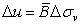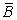Expert Modelling: Hands-on help from Rocscience Engineers. Register Here

# Excess Pore Pressure

The Excess Pore Pressure tab in the Material Properties dialog, will only be available if the Excess Pore Pressure option is selected on the Groundwater page of the Project Settings dialog.

Excess pore pressure refers to a sudden increase in pore pressure within a soil due to rapidly applied loading conditions (undrained loading). Materials with low permeability such as clays, may exhibit this behaviour. With the so-called "B-bar" method, the change in pore pressure is assumed to be directly proportional to the change in vertical stress. The excess pore pressure is given by:Eqn.1

where(B-bar) is the overall pore pressure coefficient for a material ( Skempton (1954) ). The change in vertical stress can be due to:

• the weight of added layers of material
• or a combination of these factors

as specified by the user. Only the vertical component of loads can affect the pore pressure.

For example, if an embankment is constructed over a clay foundation, this can create a temporary condition of "excess pore pressure" within the clay. Due to low permeability, the excess pore pressure within the clay cannot readily dissipate. A non-steady state situation then exists, in which the elevated pore pressure can lead to lower safety factors, and possible failure. Note that the term "rapid" loading is relative to the ability of a material to dissipate pore pressure, and may refer to time periods of days or even weeks.

The final pore pressure which is used in the stability calculations is equal to the Initial Pore Pressure + Excess Pore Pressure.

• The Initial Pore Pressure is determined by the Groundwater Method and the Water Parameters used for the initial pore pressure calculation (e.g. Water Table).
• The Excess Pore Pressure is calculated from the B-bar coefficient and the change in vertical stress.

## B-Bar

To calculate Excess Pore Pressure for a material, enter a B-bar coefficient greater than zero. The value of B-bar is usually between 0 and 1, but may be greater than 1 in some cases.

For materials which are free-draining (i.e. will not develop excess pore pressure in response to a change in vertical stress), use B-bar = 0.

Material weight creates excess pore pressure

If the Material weight creates excess pore pressure checkbox is selected for a material, then the material weight will contribute to the "change in vertical stress" used to calculate the excess pore pressure in materials which lie beneath that material in the model.

NOTE: excess pore pressure is only calculated in materials with B-bar > 0. For materials with B-bar = 0, no excess pore pressure will result.

For a tutorial which demonstrates the Excess Pore Pressure option, see Tutorial __ on the Slide3 Tutorials page.